Technical Article

# Understanding Noise and PSRR in LDOs

June 16, 2015 by Editorial Team

## The effect of noise and Power Supply Rejection Ratio (PSRR) in Low-dropout (LDO) regulators.

The effect of noise and Power Supply Rejection Ratio (PSRR) in Low-dropout (LDO) regulators.

#### Recommended Level

Intermediate

In this article, we will cover the effect of noise and Power Supply Rejection Ratio (PSRR) in Low-dropout (LDO) regulators. Let us discuss briefly what LDOs are.

#### Low-dropout Regulator

A low-dropout​or LDO​ regulator is a DC linear voltage regulator by which the output voltage can be controlled even when the input voltage supplied to it is nearly equal to the output voltage. There are two components of LDO - Power FET and a Differential Amplifier (error amplifier). Configuration of LDO can be seen in figure below: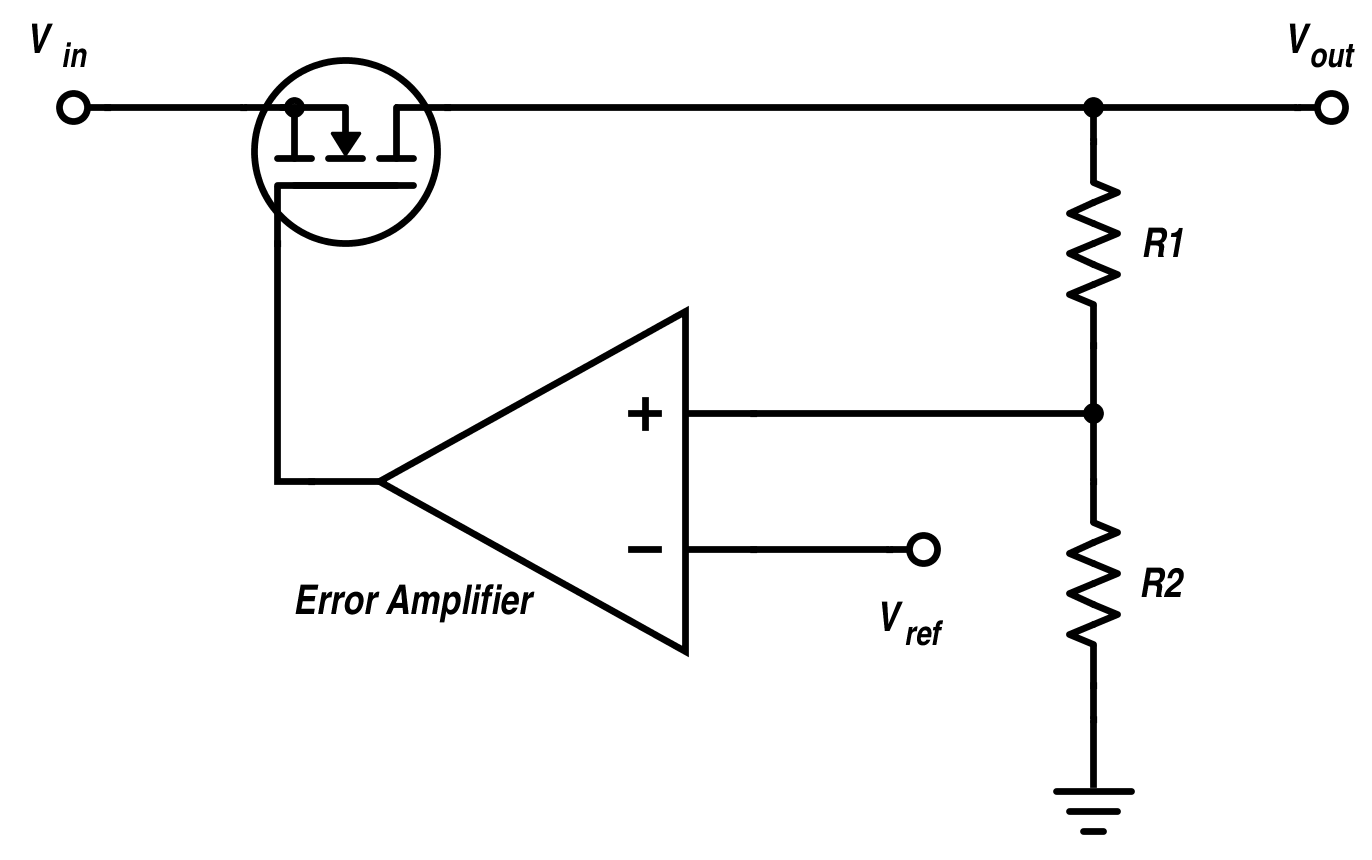#### Noise in Low-dropout Regulators (LDOs)

The noise sources in a low-dropout (LDO) regulator can be divided into two wide categories i.e. intrinsic and extrinsic. There are two major sources of intrinsic noise in LDOs:
1.    The internal reference voltage.
2.    The error amplifier.

Whereas, extrinsic noise is like the noise from a jet airplane and is passed on from a source outside the circuit.

In order to attain quiescent currents of 15 μA or less, modern LDOs manage with internal bias currents that are of a few tens of nano amps.

#### Reducing Noise in LDO

The two main methods to reduce the LDO noise are:
1.    Filtering the reference
2.    Reducing the noise gain of the error amplifier

In some LDOs, an external capacitor is used to filter the reference. It is realism that, to attain their low noise condition, many so-called ultralow noise LDOs require an external noise attenuation capacitor. Unluckily, it is not possible to get reduced output noise for fixed output LDOs because there is no right of entry to the feedback node. If the error amplifier contributes more in noise than the contribution of the reference, then overall noise of LDO can be reduced by dropping the noise gain of the error amplifier.

The only way to conclude rather an error amplifier is the main noise contributor or not, is by comparing the noise of the fixed version with the variable versions of a particular LDO. If the fixed LDOs have a smaller amount of noise than the variable LDOs, then we can say that the error amplifier is the major source of noise.

The figure shows a 2.5 V output modifiable LDO with R1, R2, R3 and C1 that are exterior components.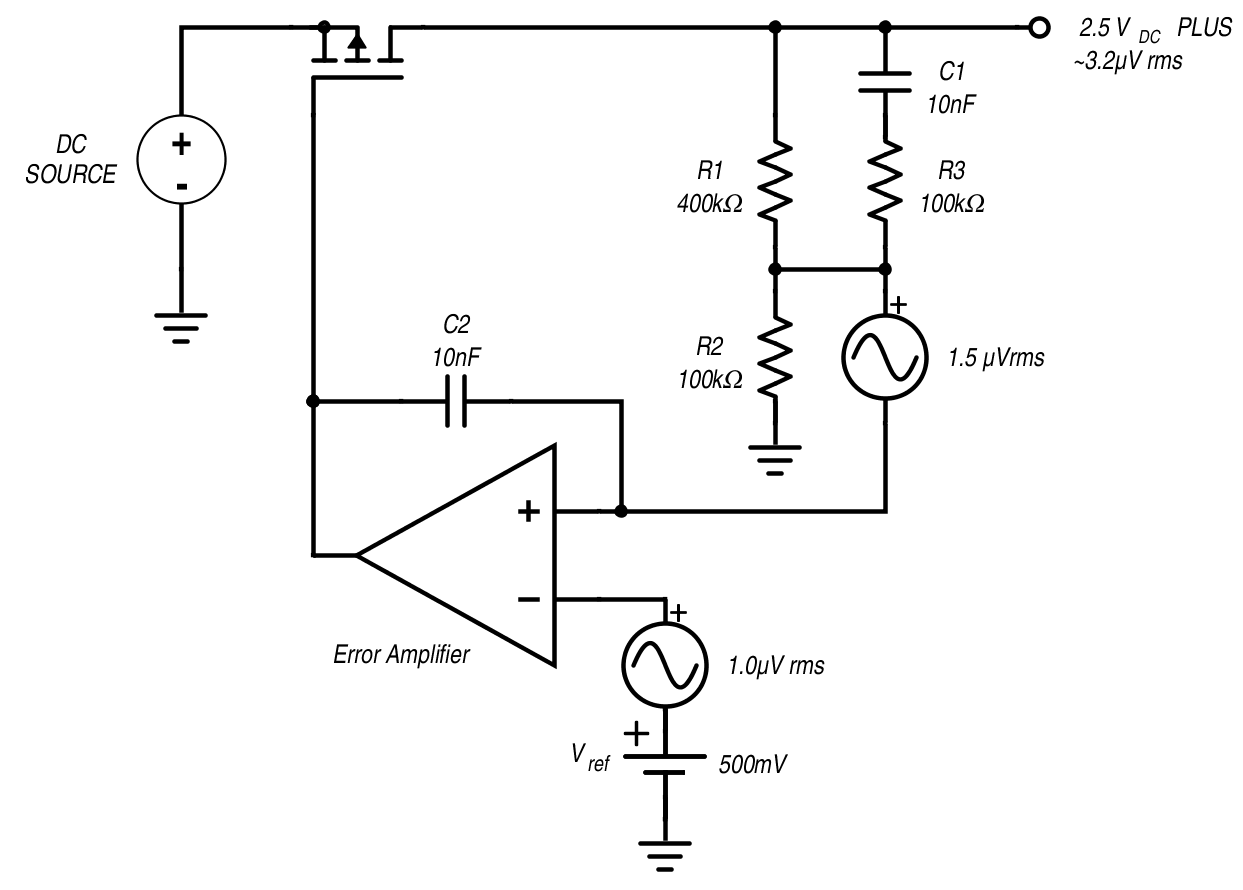R3 is used to place the high frequency gain of the amplifier up to 1.5× to 2×. Whereas, C1 is used to set the low frequency zero of the noise reduction system (C1, R1, and R3) between 10 Hz and 100 Hz to make sure that the noise is reduced up to 1/f.

The result of the noise reduction (NR) network on the noise spectral density of a high voltage adaptable LDO can be seen in graph below.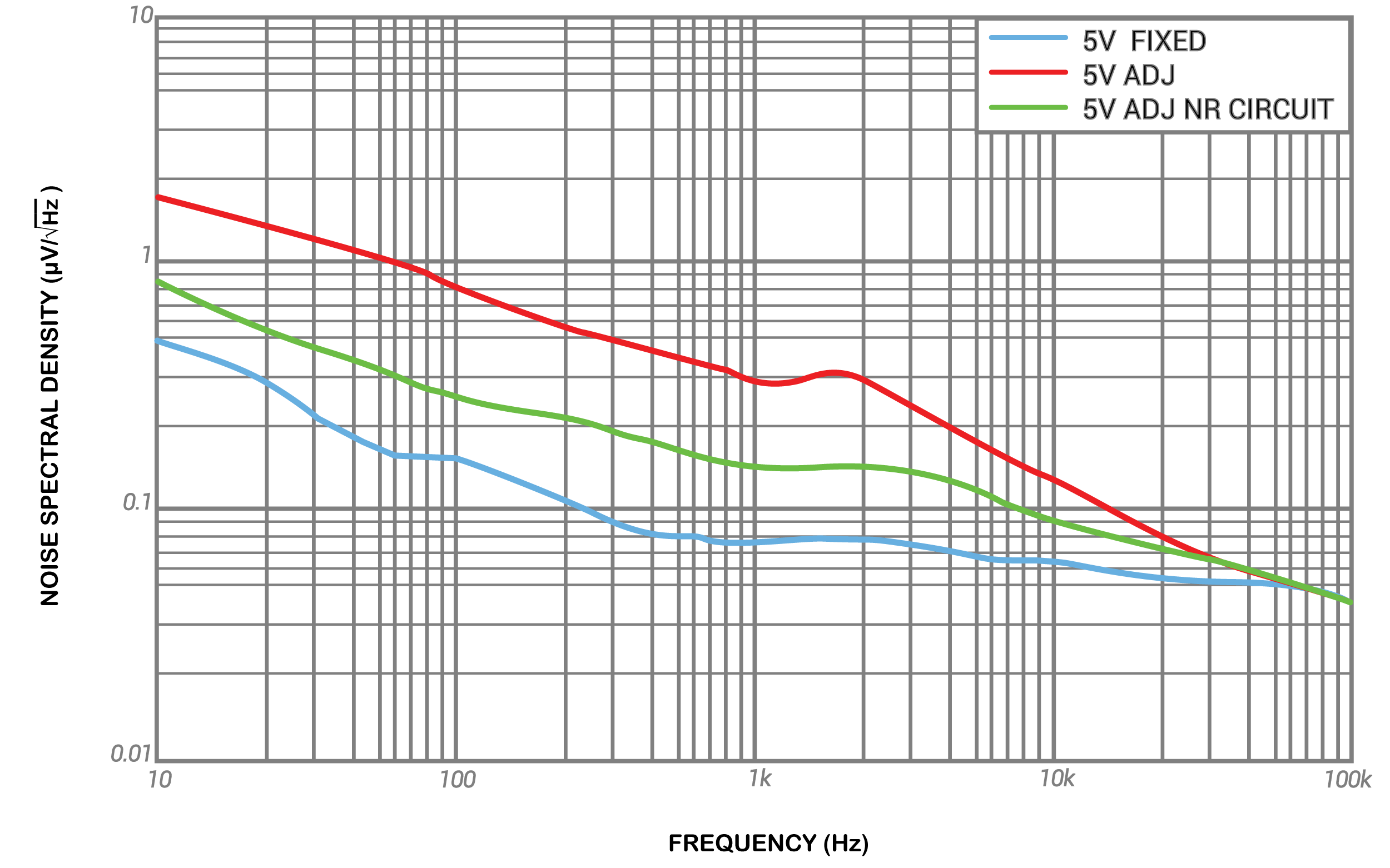In the graph above, it can be seen that there is a progress of about a factor of three (~10 dB) in the noise performance between 20 Hz and 2 kHz.

#### Power Supply Rejection Ratio in LDOs

PSRR stands for “Power Supply Rejection Ratio” and it has become progressively more important parameter in modern system on chip (SoC) design because of increased level of integrating things.

The PSRR is a ratio between two transfer functions:
•    Transfer function of the supply node to the output node i.e. (Asupply(ω))
•    Transfer function of the input node to output node A(ω). A(ω) is also called as open loop transfer function.

$$PSRR(ω) = 20 · log [A(ω) /Asupply(ω)] [dB]$$

where,

1/ Asupply(ω) is the reciprocal of the power supply gain which is known as PSR.

It is obvious from the above equation that PSRR is proportional to A(ω) and inversely proportional to Asupply(ω). As a result, if Asupply(ω) decreases and open-loop gain A(ω) is increased, the PSRR will be increased. PSRR is basically the ability of LDO’s to reject ripples that occur at input side. In an ideal LDO, DC frequency would be the only output voltage. However, the error amplifier does not have perfect functionality due to small spikes which occur at high frequencies. By taking into account ripples, PSRR is expressed as below:
PSRR=20 xlog RippleinputRippleoutput

#### Practical Example

An LDO that has:

PSRR= 55 dB

Frequency= 1 MHz

Input ripple = 1mV

It can attenuate a 1 mV at this frequency to just 1.78 µV at the output. So, there is 6dB increase in PSRR, which is equals to an increase in attenuation by a factor of 2.

Most LDOs have comparatively high PSRR at lower frequencies normally 10 Hz – 1 kHz. The LDO having high PSRR over a wide band can reject very high frequency noise same like noise arising from a switcher.
PSRR fluctuates over some parameters like frequency, temperature, current, output voltage, and the voltage differential. PSRR should be a negative value because it is used to calculate rejection. However, the graph shows it as positive number so that a top number in graph denotes higher noise rejection.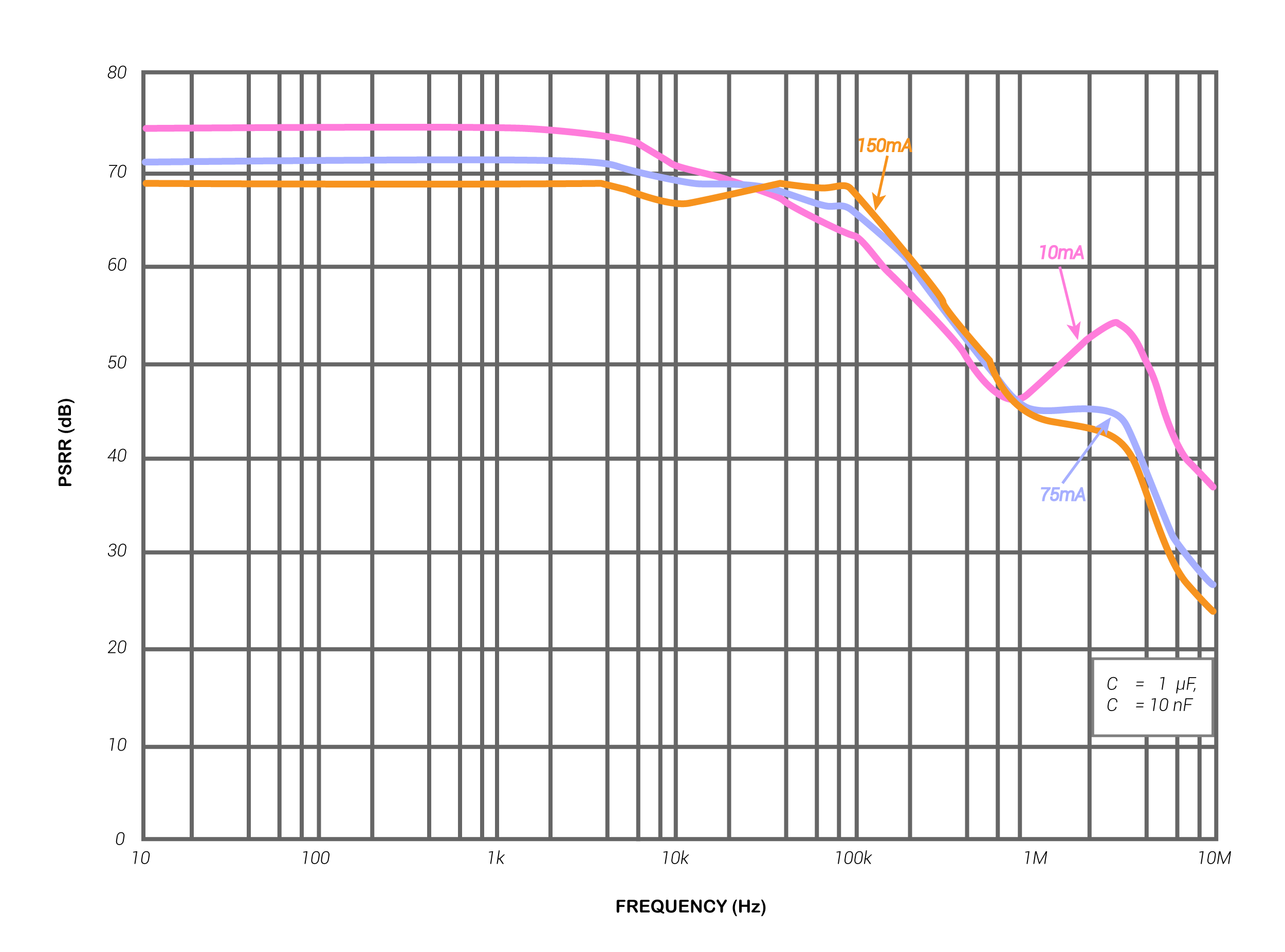#### Measuring PSRR of LDO

There are different methods of measuring PSRR of an LDO:

1.    Measurement of  PSRR by using LC summing node:

The basic method of measuring PSRR of LDO is shown in figure below.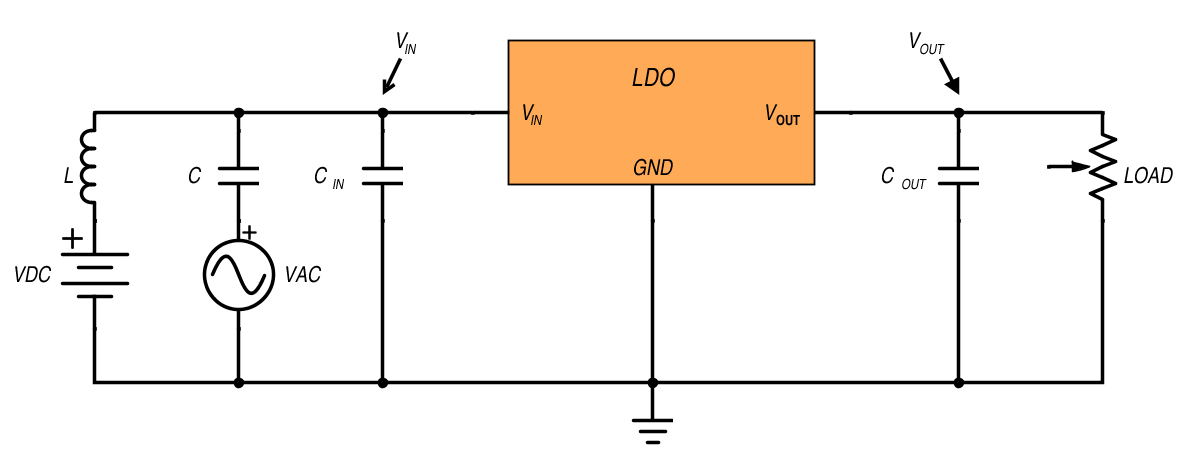In this method, two voltages (DC and AC) are added up together and applied at the input terminal of the LDO. Operating point bias voltage is VDC and VAC is the noise source. In figure above, Capacitor C is used to prevent VAC from shorting VDC and inductor L prevents VDC from                shorting noise source.

To measure PSRR in low frequency is determined by a high pass filter created by inductor L and capacitor C. The 3dB point of this filter is determined by:
$$Fmin= 12ΠLC$$
Measuring PSRR becomes difficult when frequencies are obtained below the 3dB point and they start to attenuate.

2.    Measuring PSRR using summing amplifier

In order to get improved measurement of PSRR, another method is described in which a high-bandwidth amplifier is used as summing node to insert the signals and as a result, it provides isolation between VAC and VDC. This method can be seen in the figure below: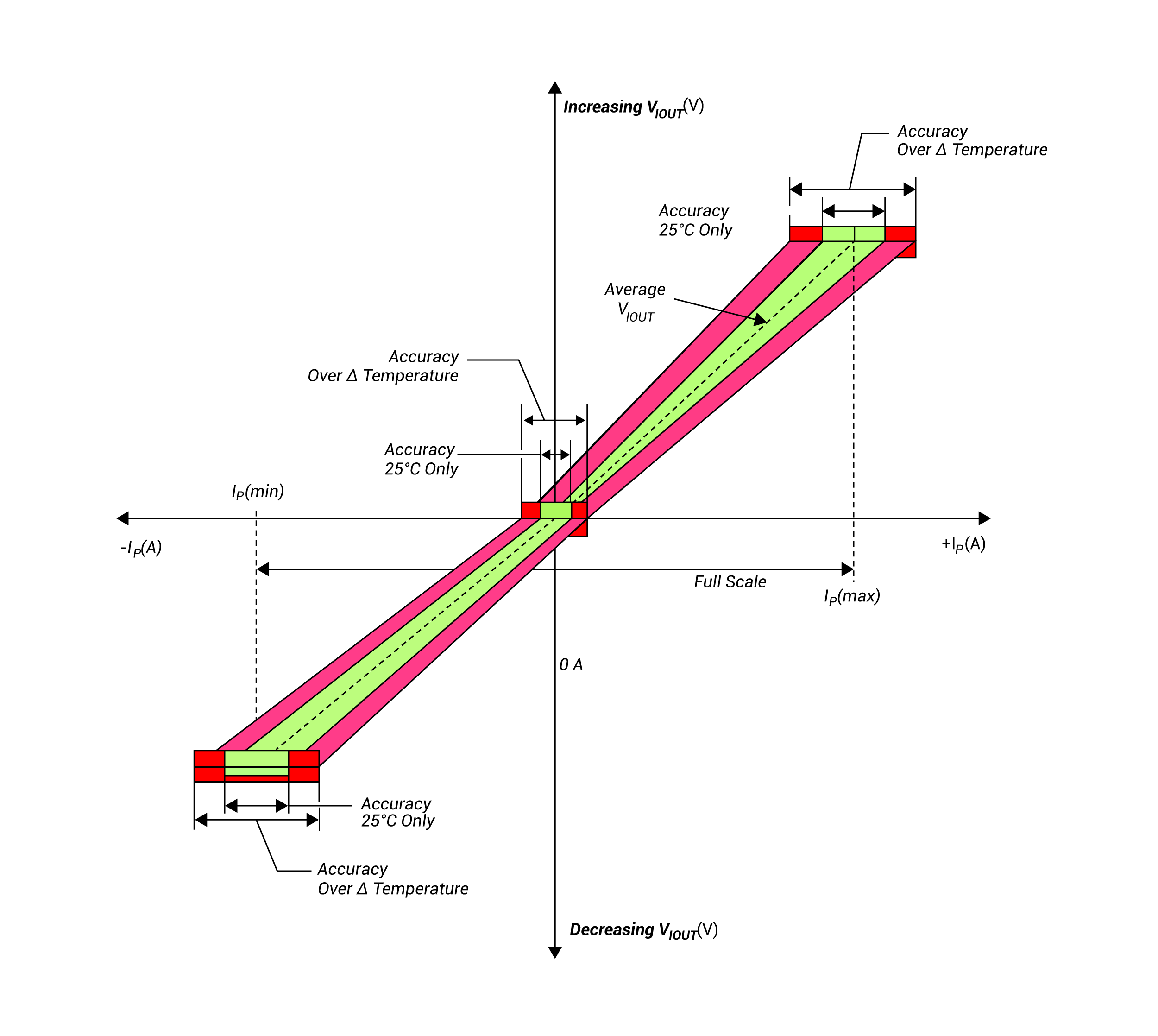In this method, PSRR is measured under no load condition.

The following factors have to be kept in mind while measuring PSRR, when this method is used.
1.    The input capacitor could be the reason of high-speed amplifier to go in an unstable condition so; this capacitor should be removed before measurement of PSRR.
2.    In order to lessen the inductance effect, Vin and Vout should be measured with either scope or network analyzer at once.
3.    Long wires add inductance and leave an impact on the results. That’s why, test set-up should not have any long wire.
4.    Following conditions should be considered while selecting the values of AC and DC inputs:
•    VAC (max) + VDC < VABS (max) of LDO
•    VDC – VAC > VUVLO of LDO

Best results will be obtained if:
•    VDC–VAC > Vout + Vdo + 0.5
Where:
Vout is the output voltage of the LDO
Vdo is the particular drop out voltage at the operating point.
5.    The result of the amplifier will start to attenuate the VAC signal at very high frequencies applied to the LDO.
6.    MOSFET output impedance is inversely proportional to the drain current so it causes open-loop output impedance of LDO to decrease, with increase in load current and lowering gain.